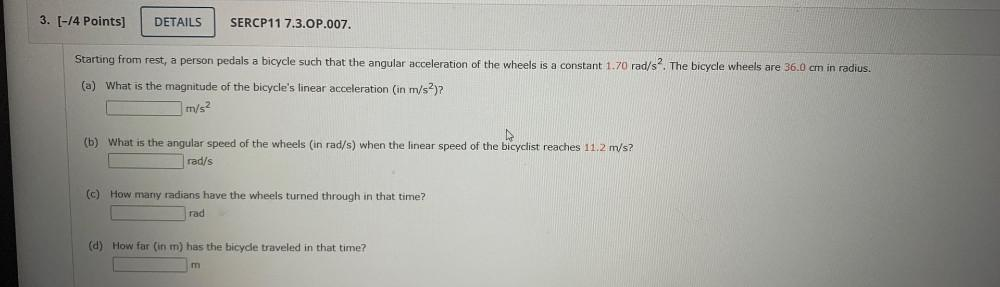Question:

# Starting from rest, a person pedals a bicycle such that the angular acceleration of the wheels is a constant 1.70 rad/s². The bicycle wheels are 36.0 cm in radius. (a) What is the magnitude of the biStarting from rest, a person pedals a bicycle such that the angular acceleration of the wheels is a constant 1.70 rad/s². The bicycle wheels are 36.0 cm in radius. (a) What is the magnitude of the bicycle's linear acceleration (in m/s²)? (b) What is the angular speed of the wheels (in rad/s) when the linear speed of the bicyclist reaches 11.2 m/s? (c) How many radians have the wheels turned through in that time? (d) How far (in m) has the bicycle traveled in that time?